# Q17. A metallic sphere of mass 1kg and radius 5 cm is welded to the end...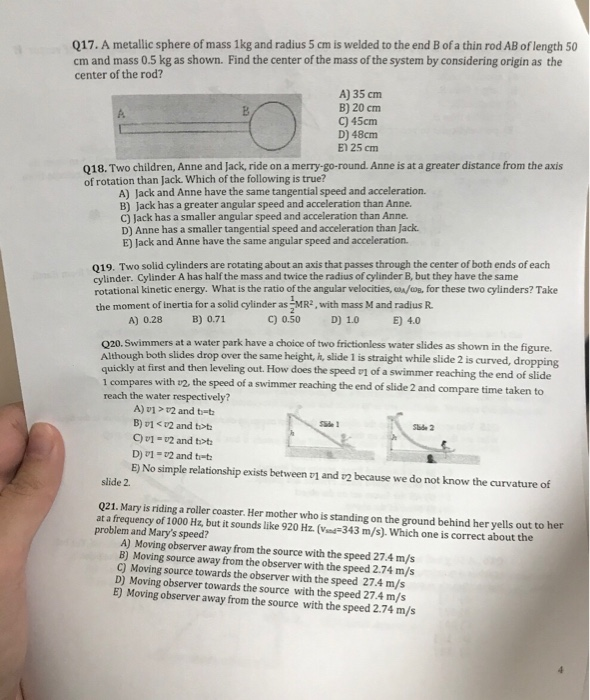Q17. A metallic sphere of mass 1kg and radius 5 cm is welded to the end B of a thin rod AB of length 50 cm and mass o.5 kg as shown. Find the center of the mass of the system by considering origin as the center of the rod? A) 35 cm B) 20 cm C) 45cm D) 48cm E1 25 cm A. Q18. Two children, Anne and Jack, ride on a merry-go-round. Anne is at a greater distance from the axis of rotation than Jack. Which of the following is true? A) Jack and Anne have the same tangential speed and acceleration. B) Jack has a greater angular speed and acceleration than Anne. C) Jack has a smaller angular speed and acceleration than Anne D) Anne has a smaller tangential speed and acceleration than Jack. E) Jack and Anne have the same angular speed and acceleration. 019. Two solid cylinders are rotating about an axis that passes through the center of both ends of each cylinder. Cylinder A has half the mass and twice the radius of cylinder B, but they have the same rotational kinetic energy. What is the ratio of the angular velocities,B,for these two cylinders? Take the moment of Inertia for a solid cylinder as MR2, with mass M and radius R. A) 0.28 B) 071 c) 0.50 D) 10 4.0 020. Swimmers at a water park have a choice of two frictionless water slides as shown in the figure. Although both slides drop over the same height, h, slide 1 is straight while slide 2 is curved, d ropping quickly at first and then leveling out. How does the speed v1 of a swimmer reaching the end of slide compares with v2, the speed of a swimmer reaching the end of slide 2 and compare time taken to reach the water respectively? A)v1 >v2 and t-t2 B) v1 < 2 and tt C)v1-v2 and tot D) 1 v2 and t-t E) No simple relationship exists between v1 and v2 because we do not know the curvature of slide 2. Q21. Mary is riding a roller coaster. Her mother who is standing on the ground behind her yells out to her at a frequency of 1000 Hz, but it sounds like 920 Hz. V 343 m/s). Which one is correct about the problem and Mary's speed? A) Moving observer away from the source with the speed 27.4 m/s B) Moving source away from the observer with the speed 2.74 m/s C) Moving source towards the observer with the speed 27.4 m/s D) Moving observer towards the source with the speed 27.4 m/s E) Moving observer away from the source with the speed 2.74 m/s

18). B

19). B

20). C

21). A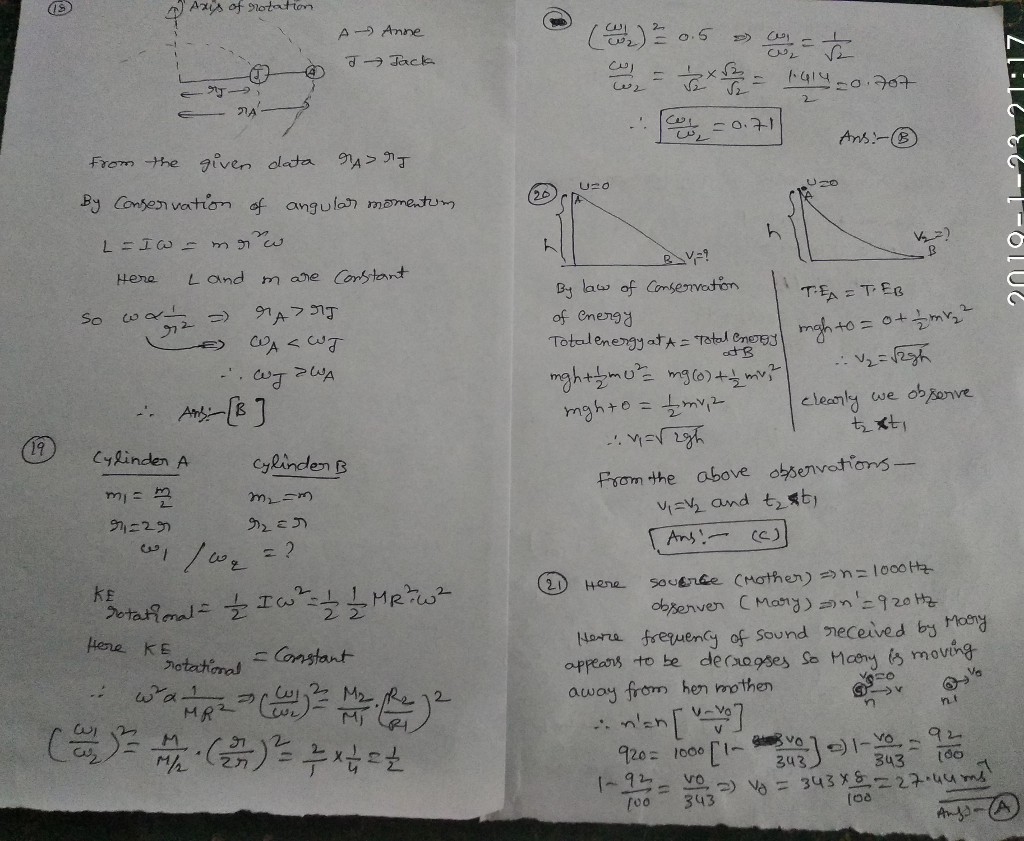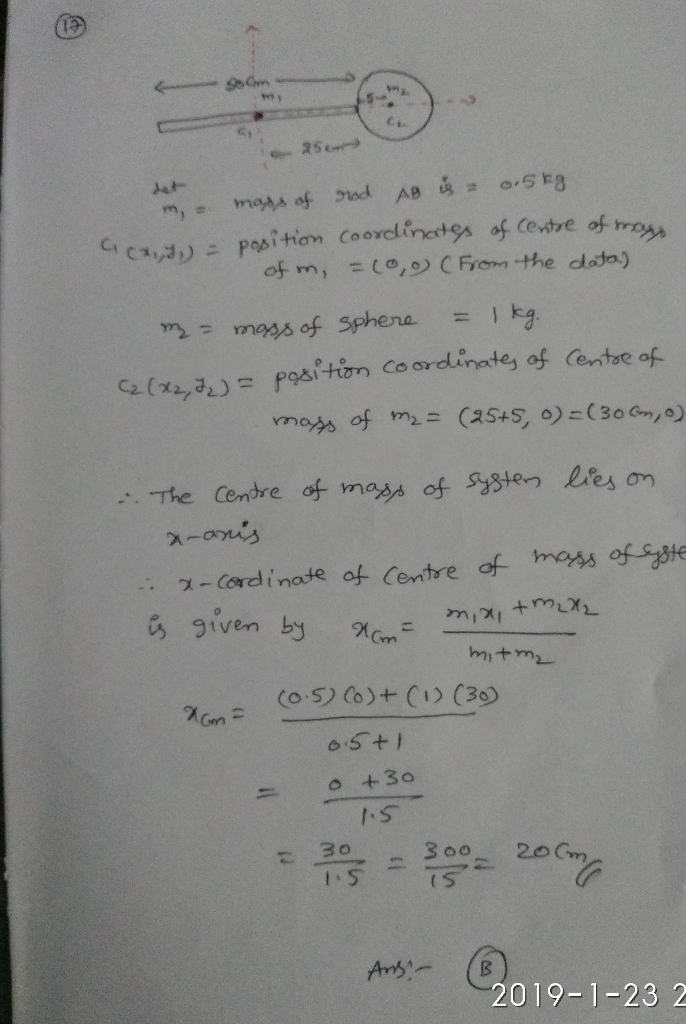#### Earn Coin

Coins can be redeemed for fabulous gifts.

Similar Homework Help Questions
• ### Q17. A metallic sphere of mass 1kg and radius 5 cm is welded to the end...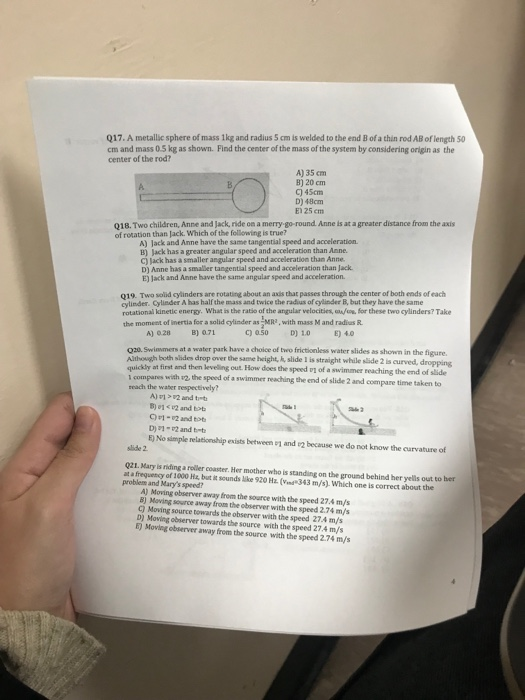Q17. A metallic sphere of mass 1kg and radius 5 cm is welded to the end B of a thin rod AB of length 50 cm and mass 0.5 kg as shown. Find the center of the mass of the system by considering origin as the center of the rod? A) 35 cm B) 20 cm C) 45cm D) 48cm E) 25 em Q18. Two children, Anne and Jack, ride on a merry go-round Anne is at a greater distance...

• ### Q21. Mary is riding a roller coaster. Her mother who is standing on the ground behind...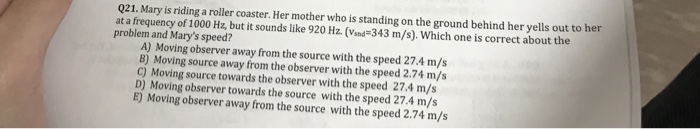Q21. Mary is riding a roller coaster. Her mother who is standing on the ground behind her yells out to her at a frequency of 1000 Hz, but it sounds like 920 Hz. (Vsnd 343 m/s). Which one is correct about the problem and Mary's speed? A) Moving observer away from the source with the speed 27.4 m/s B) Moving source away from the observer with the speed 2.74 m/s c) Moving source towards the observer with the speed 27.4...

• ### Q21. Mary is riding a roller coaster. Her mother who is standing on the ground behind...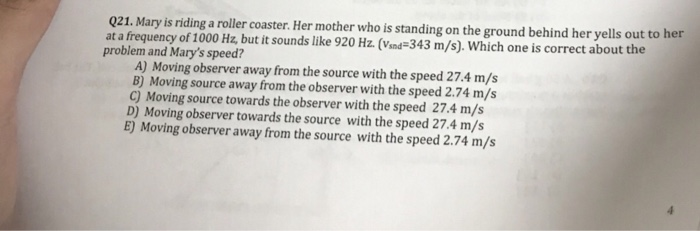Q21. Mary is riding a roller coaster. Her mother who is standing on the ground behind her yells out to her at a frequency of 1000 Hz, but it sounds like 920 Hz. (Vind343 m/s). Which one is correct about the problem and Mary's speed? A) Moving observer away from the source with the speed 27.4 m/s B) Moving source away from the observer with the speed 2.74 m/s C) Moving source towards the observer with the speed 27.4 m/s...

• ### 1. A solid cylinder has a mass of 5 kg and a radius of 30 cm. A torque of 40 Nm is applied to the cylinder. What will be...

1. A solid cylinder has a mass of 5 kg and a radius of 30 cm. A torque of 40 Nm is applied to the cylinder. What will be its angular acceleration? a) 37.5 rad/s2 b) 59.3 rad/s2 c) 89.0 rad/s2 d) 178 rad/s2 2. When an object is in static equilibrium a) the net force on it is zero b) the net torque on it is zero c) the net force and net torque are zero d) not enough...

• ### 19 pplese C) J D) A cas dgreater angular speed and acceleration than Anne. ack has...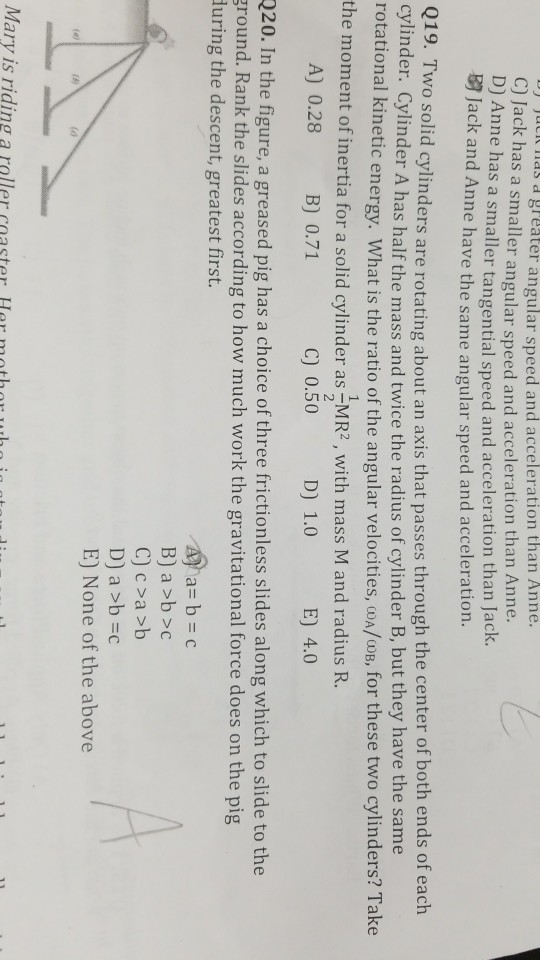19 pplese C) J D) A cas dgreater angular speed and acceleration than Anne. ack has a smaller angular speed and acceleration than Anne. nne has a smaller tangential speed and acceleration than Jack. B2 ack and Anne have the same angular speed and acceleration. 019. Two solid cylinders are rotating about an axis that passes through the center of both ends of each cylinder. Cylinder A has half the mass and twice the radius of cylinder B, but they...

• ### A solid cylinder has length L = 50.0 cm, radius R = 40.0 cm, and mass...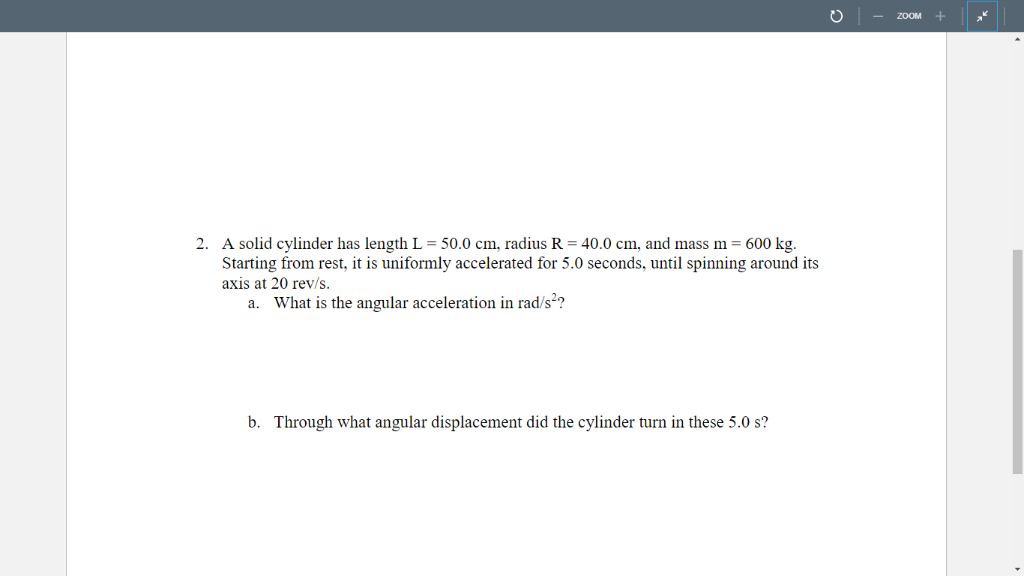A solid cylinder has length L = 50.0 cm, radius R = 40.0 cm, and mass m = 600 kg. Starting from rest, it is uniformly accelerated for 5.0 seconds, until spinning around its axis at 20 rev/s. a. What is the angular acceleration in rad/s2 ? b. Through what angular displacement did the cylinder turn in these 5.0 s? ZOOM 40.0 cm, and mass m = 600 kg 2. A solid cylinder has length L = 50.0 cm, radius...

• ### 1) A solid sphere of radius = 10 cm and mass = 2 kg is going...

1) A solid sphere of radius = 10 cm and mass = 2 kg is going down an inclined plane of height = 5 m. The angle of the inclined plane = 35 degrees. a) What is the final Total kinetic energy of the sphere? 98J b) Calculate the final Vcm and angular of the sphere and the Total velocity at the top point. Vcm=8.37m/s w=83.67 rads/s Vt=16.73m/s c) What are the final Linear (translational) and Rotational kinetic energies of...

• ### A uniform, solid sphere of radius 3.75 cm and mass 1.25 kg starts with a purely...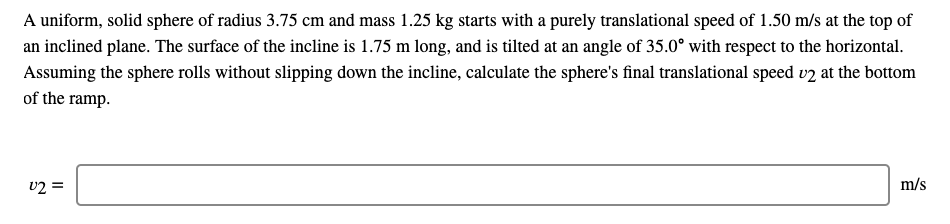A uniform, solid sphere of radius 3.75 cm and mass 1.25 kg starts with a purely translational speed of 1.50 m/s at the top of an inclined plane. The surface of the incline is 1.75 m long, and is tilted at an angle of 35.0° with respect to the horizontal. Assuming the sphere rolls without slipping down the incline, calculate the sphere's final translational speed v2 at the bottom of the ramp. v2 = m/s

• ### A uniform, solid sphere of radius 4.00 cm and mass 4.50 kg starts with a purely...

A uniform, solid sphere of radius 4.00 cm and mass 4.50 kg starts with a purely translational speed of 2.25 m/s at the top of an inclined plane. The surface of the incline is 2.75 m long, and is tilted at an angle of 33.0" with respect to the horizontal. Assuming the sphere rolls without slipping down the incline, calculate the sphere's final translational speed v2 at the bottom of the ramp. v2 = _______ m/s

• ### help 13.Two spheres of mass M and radius R are both released from rest at the...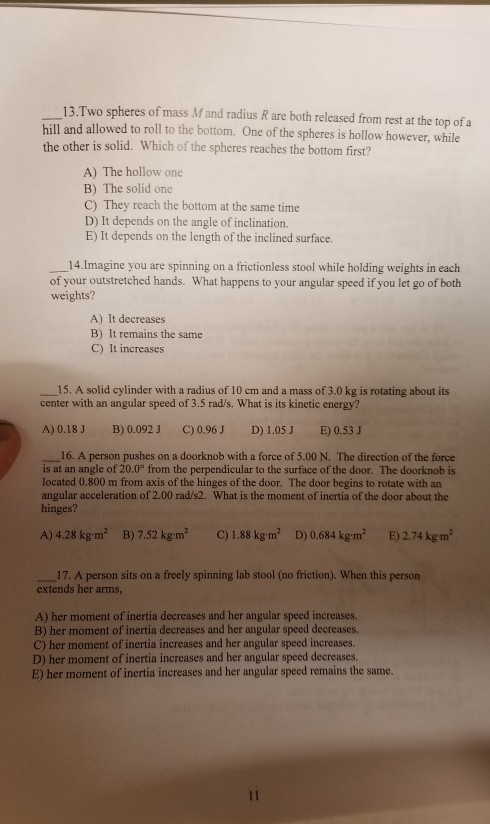help 13.Two spheres of mass M and radius R are both released from rest at the top of a hill and allowed to roll to the bottom. One of the spheres is hollow however, while the other is solid. Which of the spheres reaches the bottom first? A) The hollow one B) The solid one C) They reach the bottom at the same time D) It depends on the angle of inclination. E) It depends on the length of the...